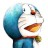# 杰拉斯的博客

## [ACM_NYOJ_10]Skiing（深度优先搜索&动态规划）杰拉斯 | 时间：2012-04-17, Tue | 9,623 views

### Skiing

#### 描述

Michael喜欢滑雪百这并不奇怪， 因为滑雪的确很刺激。可是为了获得速度，滑的区域必须向下倾斜，而且当你滑到坡底，你不得不再次走上坡或者等待升降机来载你。Michael想知道载一个区域中最长底滑坡。区域由一个二维数组给出。数组的每个数字代表点的高度。下面是一个例子：

1 2 3 4 5
16 17 18 19 6
15 24 25 20 7
14 23 22 21 8
13 12 11 10 9

```1
5 5
1 2 3 4 5
16 17 18 19 6
15 24 25 20 7
14 23 22 21 8
13 12 11 10 9
```

```25
```

#### 来源

NYOJ10

```#include<stdio.h>
#include<memory.h>
int R, C;
int a;	//各点高度
int f;	//各点最大滑雪长度
inline int max(int a, int b){	//inline表示编译时直接嵌入至调用处，节省调用函数的时间
return a>b?a:b;
}
inline int max(int a, int b, int c, int d){
return max(max(a, b), max(c, d));
}
int dfs(int row, int col, int h){	//递归深搜
if(row < 1 || row > R || col < 1 || col > R || h <= a[row][col])	//超出范围或上一点高度低于该点高度
return 0;	//则返回0
if(f[row][col] >= 0)	//如果已经搜索过
return f[row][col];	//则直接返回该点最大化学长度
f[row][col] = max(dfs(row - 1, col, a[row][col]), dfs(row, col + 1, a[row][col]), dfs(row + 1, col, a[row][col]), dfs(row, col - 1, a[row][col])) + 1;	//动规，当前最大滑雪长度为四周比该点低的最大滑雪长度加1
return f[row][col];
}
int main(){
int T, i, j;
scanf("%d", &T);
while(T--){
int max = 0;
memset(f, -1, sizeof(f));
scanf("%d%d", &R, &C);
for(i = 1; i <= R; ++i){
for(j = 1; j <= R; ++j){
scanf("%d", &a[i][j]);
}
}
for(i = 1; i <= R; ++i){
for(j = 1; j <= R; ++j){
int num = dfs(i, j, 0xffffff);	//通过16进制0xffffff方便地给出一个足够大的int型
if(max < num)
max = num;
}
}
printf("%d\n", max);
}
return 0;
}
```